Business relationships with the customers are not static – they change over time. It is crucial for the company to understand these dynamic processes. The customer relationship management (CRM) is a huge area that encompasses multiple practices, directions, and approaches related to customer interactions with the business. One of the central directions is the Customer Lifecycle Value (CLV), which is the topic of this session. The first approach that we will study is based on heuristics, where we do not use any automatic methods, but a fairly simple analysis in order to calculate Customer Lifetime Value (CLV). Then, we will learn how to use simple and multiple linear regressions in the context of CRM. We will follow machine learning practices discussed during the lecture.

Let’s install & load the required packages:

install.packages("tidyverse")
install.packages("data.table")
library("tidyverse")
library("data.table")

and the dataset:

dt_raw <- read.csv(file.choose())
head(dt_raw, 3)
##   transaction_id transaction_date customer_id amount
## 1              1       2012-09-04           1  20.96
## 2              2       2012-05-15           2  10.87
## 3              3       2014-05-23           2   2.21
• transaction_id - unique ID of some transaction;
• transaction_date - date when transaction was executed;
• customer_id - unique ID of the customer;
• amount - total amount of money the were transfered.

## Customer Lifecycle Value

Our goal here is to calculate CLV, which is the cash flow attributed to the customer during the entire relationship with the company. CLV is a metric that can be used for many different purposes:

• estimation of the customer value in order to target the most valuable ones
• reference for the markieting campaigns: how much to spend or whether to spend?
• and monitoring the impact of such campaigns
• measurement of customer loyalty
• optimization of resource allocation
• etc..

There are many methodologies of CLV, which can be very complex and include all the details taking into account all the spendings. More generally, CLV can be divided into two broad categoris: historical CLV and predictive CLV. Let us take the simple approach and calculate the historical value.

summary(dt_raw)
customers_stat <- dt_raw %>%
group_by(customer_id) %>% # group intial dataset by customers
summarise(transaction_per_customer = n(), # calculates total number of transactions for each customer
amount_per_customer = sum(amount), # adds up all amounts for each customer
amount_per_transaction = amount_per_customer/transaction_per_customer)
# calculate statistics on the customers

summarise_all(customers_stat[,-1], mean) # calculate averages (wrt customer) 
## # A tibble: 1 x 3
##   transaction_per_customer amount_per_customer amount_per_transaction
##                      <dbl>               <dbl>                  <dbl>
## 1                     4.18                33.7                   7.95
ggplot(dt_raw, aes(x=amount)) +
geom_histogram(fill='#88d8b0', color='white') # histogram of transactional amounts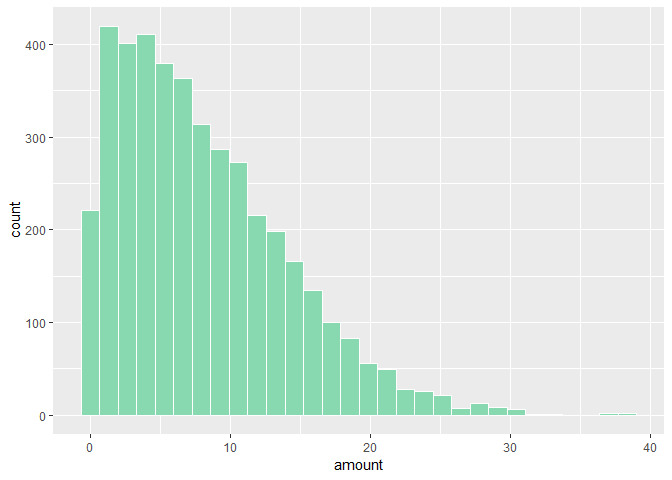Let’s keep the raw dataset unchanged and create a new dataset, where we start to collect required features:

customers <- dt_raw %>%
group_by(customer_id) %>%
mutate(year = as.integer(substr(transaction_date, start=1, stop=4))) %>% # we take only year
mutate(min_year = as.integer(min(year)),
max_year=as.integer(max(year)),
years_active=as.integer((max_year-min_year)+1)) # years of first and last purchase of the customer and amount of years he/she was active

Let’s check the timespan of a few customers to understand the data better:

set.seed(888) # put any number for reproducibility of pseudorandom generator
customers_sample <- sample(unique(customers$customer_id), size = 10, replace = F) # take IDs of 10 random customers filter(customers, customer_id %in% customers_sample) %>% # we pick only rows where customer id is the one we sampled (operator %in% will take all the rows where the customer id matches) ggplot(aes(x=year, y=customer_id, color=as.factor(customer_id))) + geom_line() + geom_point() # y axis is not important, we just want to follow customers in time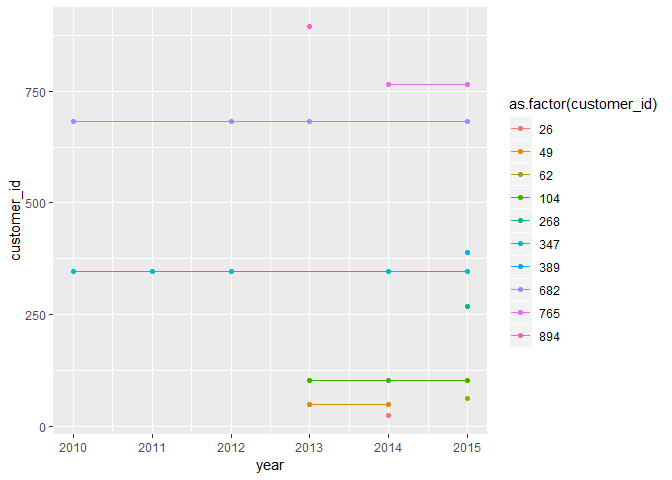We can note several important things. Namely, the customers are heterogeneous – they spend at different years, and also do not purchase every single year. Now, here we have to make a decision how to define active customers. One definition would be to consider in our calculations any customer who kept purchasing (even if in this year he did not purchase anything). Or take into account (per year basis) only a set of customers who were purchasing in the considered year. We will adopt the second approach as it will be more tricky to calculate: active_customers <- customers %>% ungroup() %>% # removing existing groups group_by(min_year, year) %>% # group customers by the starting year and the transactional years. # here we forget about customer ID and start to calculate yearly numbers summarise(customers_unique=length(unique(customer_id)), # number of unique customers for the pairs (starting year, transactional year) transactions_unique=length(unique(transaction_id)), # number of unique transactions amount = sum(amount)) %>% # amount of money spent mutate(cumulative_amount=cumsum(amount)) #cumulative amount of money spent head(active_customers,3) ## # A tibble: 3 x 6 ## # Groups: min_year  ## min_year year customers_unique transactions_un~ amount cumulative_amou~ ## <int> <int> <int> <int> <dbl> <dbl> ## 1 2010 2010 172 260 2255. 2255. ## 2 2010 2011 93 177 1359. 3614. ## 3 2010 2012 104 195 1658. 5272. active_customers_table <- active_customers %>% # for nice matrix format select(min_year, year, customers_unique) %>% # you can pick any feature to display in the matrix spread(key=year, value=customers_unique) active_customers_table ## # A tibble: 6 x 7 ## # Groups: min_year  ## min_year 2010 2011 2012 2013 2014 2015 ## <int> <int> <int> <int> <int> <int> <int> ## 1 2010 172 93 104 91 103 82 ## 2 2011 NA 170 92 98 89 88 ## 3 2012 NA NA 163 109 98 90 ## 4 2013 NA NA NA 180 103 102 ## 5 2014 NA NA NA NA 155 90 ## 6 2015 NA NA NA NA NA 160 The second part is to calculate for each year the number of new customers: new_customers <- customers %>% # we have a different grouping, so it is easier to start new table ungroup() %>% group_by(min_year) %>% # grouping only by starting year summarise(new_customers=length(unique(customer_id))) # how many unique customers where each starting year? head(new_customers,3) ## # A tibble: 3 x 2 ## min_year new_customers ## <int> <int> ## 1 2010 172 ## 2 2011 170 ## 3 2012 163 Combine the two datasets: active_customers <- left_join(active_customers, new_customers, by='min_year') # adding new_customers column to active_customers head(active_customers, 3) ## # A tibble: 3 x 7 ## # Groups: min_year  ## min_year year customers_unique transactions_un~ amount cumulative_amou~ ## <int> <int> <int> <int> <dbl> <dbl> ## 1 2010 2010 172 260 2255. 2255. ## 2 2010 2011 93 177 1359. 3614. ## 3 2010 2012 104 195 1658. 5272. ## # ... with 1 more variable: new_customers <int> Now, we are ready to calculate several important measures: 1. $$\text{customer retention rate} = \text{active customers}/ \text{new customers}$$ 2. $$\text{transactions per customer} = \text{transactions}/ \text{active customers}$$ 3. $$\text{amount per transaction} = \text{amount}/\text{transactions}$$ active_customers_stat <- active_customers %>% mutate(retention = customers_unique/new_customers) %>% mutate(transaction_per_customer = transactions_unique/customers_unique) %>% mutate(amount_per_transaction = amount/transactions_unique) active_customers_stat %>% ungroup() %>% ggplot(aes(y=retention, x=year, group=min_year, color=as.factor(min_year))) + geom_point(size=3) + geom_line()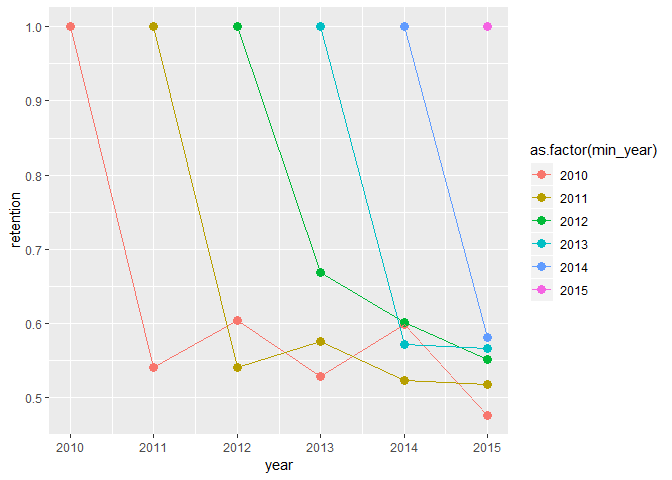active_customers_stat %>% ungroup() %>% ggplot(aes(y=as.factor(min_year), x=year)) + geom_tile(aes(fill=transaction_per_customer), colour='white') + scale_fill_gradient(low = "white", high = "steelblue")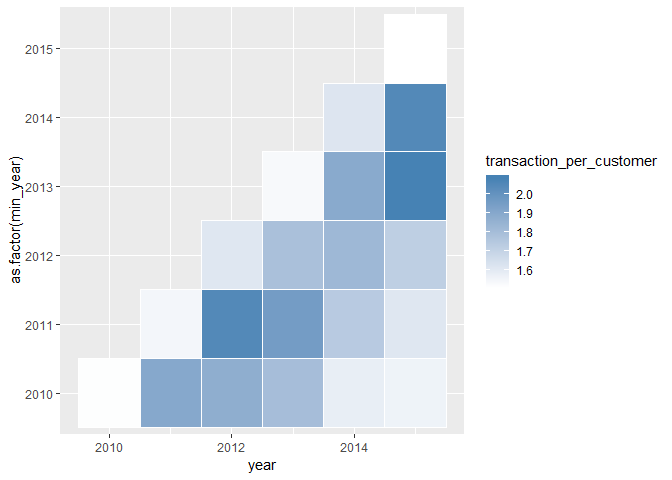Looking long enough at these numbers :), we can infer: 1. we are able to retain around 60% of the new customers for the next year 2. the transactions per customer are somewhat higher than for the first year - it is in our interest to keep customers Now we can also calculate the historical CLV, which is cumulative spending per customer over time divided by amount of new customers each year active_customers_stat <- active_customers %>% mutate(historicCLV = cumulative_amount/new_customers) historicCLV <- active_customers_stat %>% select(contains('year'), historicCLV) %>% spread(key=year, value=historicCLV) historicCLV ## # A tibble: 6 x 7 ## # Groups: min_year  ## min_year 2010 2011 2012 2013 2014 2015 ## <int> <dbl> <dbl> <dbl> <dbl> <dbl> <dbl> ## 1 2010 13.1 21.0 30.7 38.5 46.1 52.1 ## 2 2011 NA 13.2 22.1 32.1 39.4 46.2 ## 3 2012 NA NA 13.4 23.8 32.1 39.9 ## 4 2013 NA NA NA 12.1 20.1 29.1 ## 5 2014 NA NA NA NA 11.8 21.1 ## 6 2015 NA NA NA NA NA 12.0 active_customers_stat %>% ungroup() %>% ggplot(aes(x=year, y=historicCLV, color=as.factor(min_year))) + geom_point() + geom_line()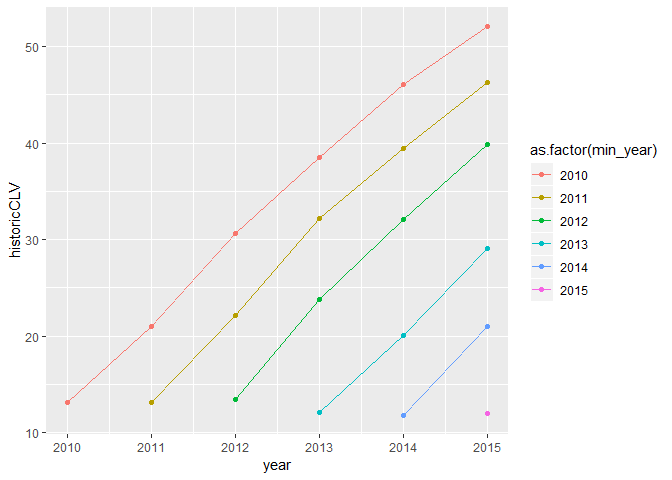If we want to know just the age of customers in months (e.g we want customers who are 12 months old considered the same instead of separate cohorts), we need to bring it to common scale: active_customers_stat <- active_customers_stat %>% ungroup() %>% mutate(months_active = ((year-min_year)+1)*12) %>% # instead of years we calculate months till start mutate(min_year = as.factor(min_year)) ggplot(active_customers_stat, aes(x=months_active, y=historicCLV, color=min_year)) + geom_point() + geom_line()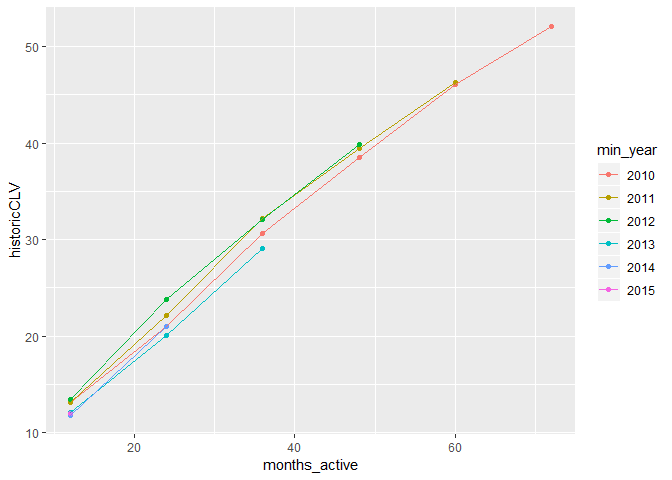The baseline CLV for the oldest customers (5 years of activity) is ~50 euros. We can also calculate weighted averages, where each cohort is weighted by the number of customers: weighted_historic_CLV <- active_customers_stat %>% mutate(vol=(historicCLV*customers_unique)) %>% group_by(months_active) %>% summarise(res=sum(vol)/sum(customers_unique)) weighted_historic_CLV ## # A tibble: 6 x 2 ## months_active res ## <dbl> <dbl> ## 1 12. 12.6 ## 2 24. 21.6 ## 3 36. 31.0 ## 4 48. 39.3 ## 5 60. 46.1 ## 6 72. 52.1 Let’s create a plot with the estimated averages of historical CLV. Also, we want to save it for further use: plot1 <- ggplot() + geom_point(data=active_customers_stat, aes(x=months_active, y=historicCLV, color=min_year)) + geom_line(data=weighted_historic_CLV, aes(x=months_active, y=res), color='grey80', size=2, alpha=0.3) plot1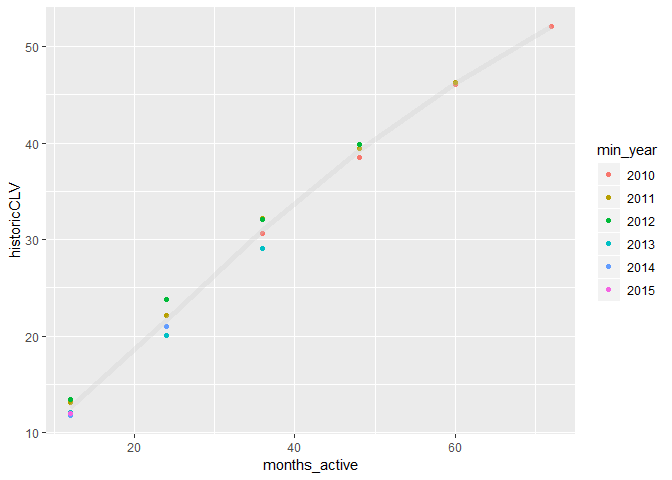## Simple Linear regression What if we want to extrapolate the curve? To predict the current values (to fit the model), and use this model for future values? # simple regression start <- Sys.time() m1 <- lm(data=weighted_historic_CLV, res~months_active) # the formula format is y~x or y~x1+x2 lm1_time <- Sys.time() - start summary(m1) # details of the models ## ## Call: ## lm(formula = res ~ months_active, data = weighted_historic_CLV) ## ## Residuals: ## 1 2 3 4 5 6 ## -1.2421 -0.1746 1.1636 1.5106 0.3965 -1.6541 ## ## Coefficients: ## Estimate Std. Error t value Pr(>|t|) ## (Intercept) 5.87048 1.32498 4.431 0.0114 * ## months_active 0.66468 0.02835 23.444 1.96e-05 *** ## --- ## Signif. codes: 0 '***' 0.001 '**' 0.01 '*' 0.05 '.' 0.1 ' ' 1 ## ## Residual standard error: 1.423 on 4 degrees of freedom ## Multiple R-squared: 0.9928, Adjusted R-squared: 0.991 ## F-statistic: 549.6 on 1 and 4 DF, p-value: 1.962e-05 weighted_historic_CLV$predictions <- predict(m1, weighted_historic_CLV) # we want to predict using the fitted model
future_predictions <- data.frame(months_active=c(12*c(7:10)), res=NA, predictions=NA) # let's create future values
future_predictions$predictions <- predict(m1, future_predictions) # make predictions for future values predictions <- rbind.data.frame(weighted_historic_CLV, future_predictions) # bind them together predictions <- gather(predictions, type, value, -months_active) # gathering for easier plotting plot2 <- plot1 + geom_line(data=predictions, aes(x=months_active, y=value, group=type, linetype=type, color=type)) plot2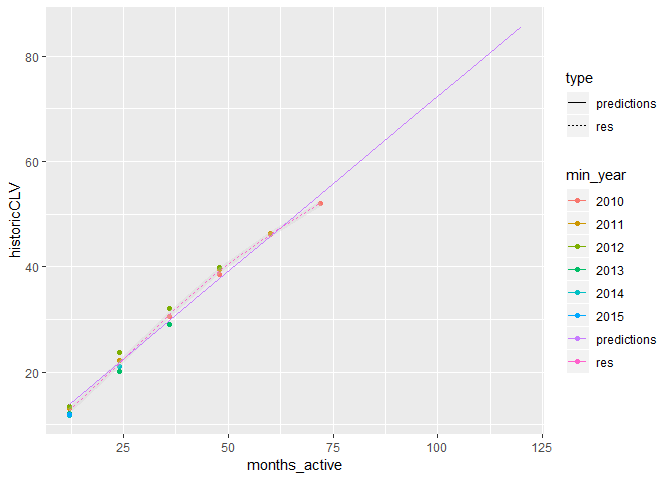## Multiple linear regression Now, what if we are more ambitious? Our desire is not just to fit general function, but to predict amount spent for each customer. Can we do that? Depends on the data. Assume that we performed a questionnaire upon the registration, collected some clicks on our website and also have information about proposed discounts for some of the customers: customer_survey <- read.csv(file.choose()) head(customer_survey, 3) ## customer_id gender age discount_proposed clicks_in_eshop ## 1 1 1 71 0 1 ## 2 2 1 42 1 3 ## 3 3 1 49 1 3 Next step is to think, how to plan our experiment. What we want to predict? We decided to predict for all customers who made transactions in their first year ($$t_{0}$$), what would be the amount of money spent next year ($$t_{1}$$). For that reason we will discard: • all customers who do not have the information about $$t_{1}$$ • all the information about future years $$t_{k}$$, where $$k>1$$ Note that we want to train generalized model : we do train the model on customers for whom we know the first and the next year, but we want to apply it on the different customers – those for whom we know only their first year, but don’t know anything about their second year (as it is in the future). For example, we would be able to apply this model using data from 2017 to predict 2018. #collect features, this one you already seen. the only difference is groupings dt_year <- dt_raw %>% mutate(year = substr(transaction_date, start=1, stop=4), year=as.integer(year)) %>% group_by(customer_id) %>% mutate(min_year = min(year), max_year=max(year), years_active=max_year-min_year) %>% # creating min, max years ungroup() %>% group_by(customer_id, year) %>% summarise(transaction_per_customer = n(), # amount of reservations in each group amount_per_customer = sum(amount), amount_per_transaction=amount_per_customer/transaction_per_customer, min_year=first(min_year), years_active=first(years_active)) # filter only those who were more than one year active dt_prep <- filter(dt_year, years_active!=0) %>% mutate(year_number=row_number()) %>% # identify what is the first, second year and so on filter(year_number %in% c(1,2)) %>% #let's take only a year old customers next year revenue. select(customer_id, transaction_per_customer, amount_per_customer, amount_per_transaction, year_number) #select features of interest # as tidyr does not allow to spread several columns, we are using dcast in data.table dt_feat_table <- dcast(setDT(dt_prep), customer_id ~ year_number, value.var=c('transaction_per_customer', 'amount_per_customer', 'amount_per_transaction')) # it is like "spread" but several columns can be spread simultaniously # discard all the features about second year (we could not know them in advance) # but keep our y - we want to predict next year amount per customer dt_feat_table <- select(dt_feat_table, -contains("_2"), 'amount_per_customer_2') Let’s join our transactional preprocessed data with our survey: dt_feat_table <- left_join(dt_feat_table, customer_survey, by='customer_id') dt_feat_table$gender <- as.factor(dt_feat_table$gender) dt_feat_table$discount_proposed <- as.factor(dt_feat_table$discount_proposed) # it is important to translate necessary features # into factors! Now, we divide our data on two sets: training and test. We want to train model on the data, but to validate the model we need to use test: set.seed(385) # fix the seed for reproducibility train_idx <- sample(nrow(dt_feat_table), round(nrow(dt_feat_table)/100*80,0), replace = F) # usualy data is splited in 20% of test data and 80% of train train <- dt_feat_table[train_idx,] test <- dt_feat_table[-train_idx,] Now we can use multiple variables to train our model: #model_1 <- lm(data=train[,-1], amount_per_customer_2 ~ .) start <- Sys.time() model_1 <- lm(data=train[,-1], amount_per_customer_2 ~ amount_per_customer_1 + transaction_per_customer_1 + amount_per_transaction_1 + gender + age + discount_proposed + clicks_in_eshop) lm3_time <- Sys.time() - start summary(model_1) ## ## Call: ## lm(formula = amount_per_customer_2 ~ amount_per_customer_1 + ## transaction_per_customer_1 + amount_per_transaction_1 + gender + ## age + discount_proposed + clicks_in_eshop, data = train[, ## -1]) ## ## Residuals: ## Min 1Q Median 3Q Max ## -11.3431 -3.0884 -0.2934 2.4622 23.4805 ## ## Coefficients: ## Estimate Std. Error t value Pr(>|t|) ## (Intercept) -24.680597 1.627710 -15.163 < 2e-16 *** ## amount_per_customer_1 0.006294 0.055650 0.113 0.9100 ## transaction_per_customer_1 0.180761 0.519212 0.348 0.7279 ## amount_per_transaction_1 0.025182 0.085411 0.295 0.7682 ## gender1 -0.798680 0.435633 -1.833 0.0673 . ## age 0.219532 0.029756 7.378 6.44e-13 *** ## discount_proposed1 -34.479706 1.861467 -18.523 < 2e-16 *** ## clicks_in_eshop 23.111674 0.834923 27.681 < 2e-16 *** ## --- ## Signif. codes: 0 '***' 0.001 '**' 0.01 '*' 0.05 '.' 0.1 ' ' 1 ## ## Residual standard error: 4.765 on 518 degrees of freedom ## Multiple R-squared: 0.8425, Adjusted R-squared: 0.8404 ## F-statistic: 396 on 7 and 518 DF, p-value: < 2.2e-16 test$prediction_linear <- predict(model_1, newdata=test[,-1]) # predictions on a test set

prediction_quality <- function(predictions, real_values){ # I wrote this function to use different measures of accuracy
diff <- sum((real_values - predictions)^2)
mse <- diff/length(predictions)
rmse <- sqrt(mse)
mae <- sum(abs(real_values - predictions))/length(predictions)
print(paste("mean squared error is ", mse))
print(paste("root mean squared error is ", rmse))
print(paste("mean absolute error is ", mae))
print(lm3_time)
}
prediction_quality(test$prediction_linear, test$amount_per_customer_2)
##  "mean squared error is  21.9160073301019"
##  "root mean squared error is  4.68145354885658"
##  "mean absolute error is  3.61740752092865"
## Time difference of 0.005999088 secs